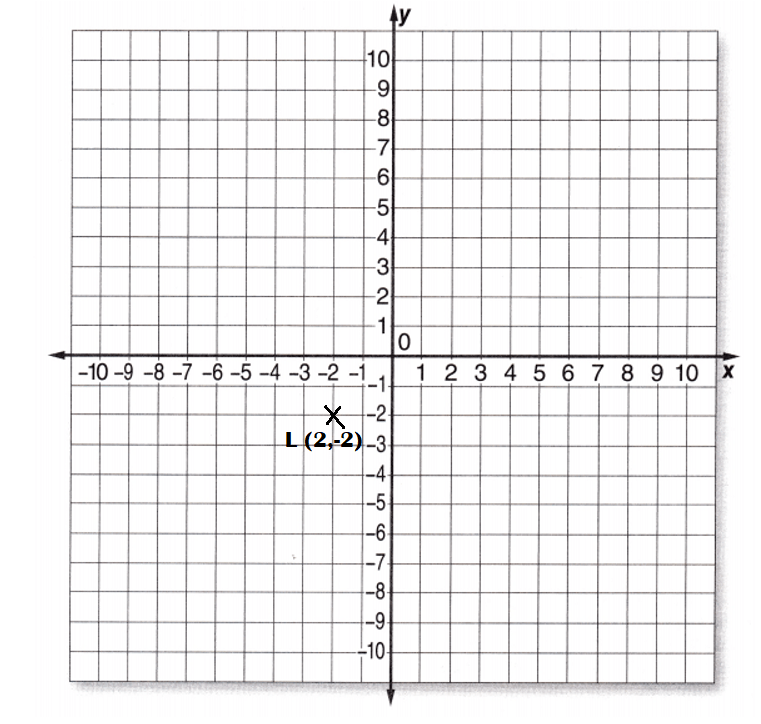# McGraw Hill Math Grade 6 Lesson 5.1 Answer Key Plotting Ordered Pairs

Practice questions available in McGraw Hill Math Grade 6 Answer Key PDF Lesson 5.1 Plotting Ordered Pairs will engage students and is a great way of informal assessment.

## McGraw-Hill Math Grade 6 Answer Key Lesson 5.1 Plotting Ordered Pairs

Exercises Give Ordered Pairs

Question 1.
Give the ordered pair for each point on the graph.Explanation:
Ordered pairs of points in the graph:
A = (1,2)
B = (3,-3)
C = (-5,-5)
D = (5,5)
E = (4,-5)
F = (1,1)
G = (-1,1)
H = (-4,4)
I = (8,8)
J = (-5,6)

Exercises
Plot Ordered Pairs

Plot the ordered pairs on the graph.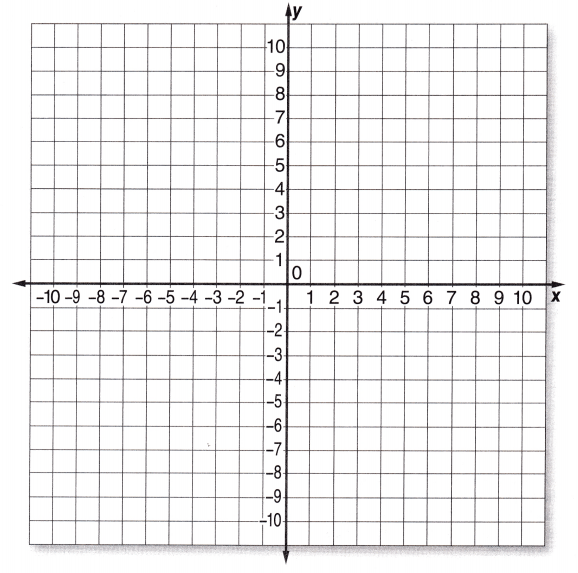Question 1.
A(2, 3)Explanation:
A(2, 3) represents (x, y) axis points in the graph.

Question 2.
B(4, -4)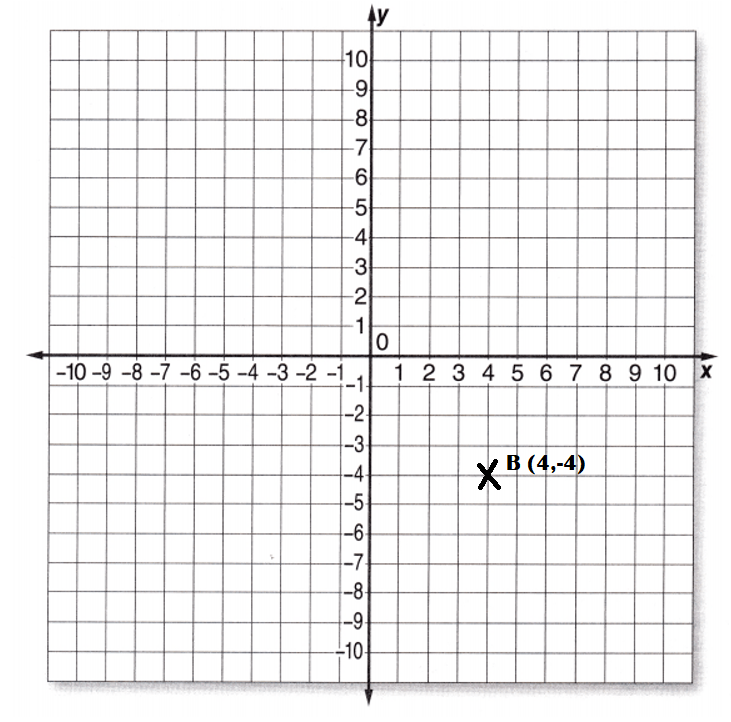Explanation:
B(4, -4)represents (x, y) axis points in the graph.

Question 3.
C(4, 4)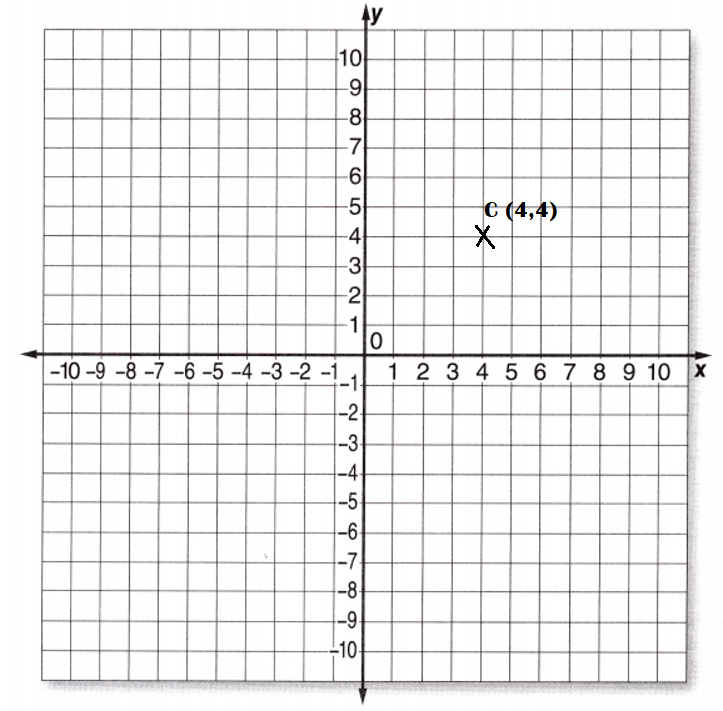Explanation:
C(4, 4) represents C(x, y) axis points in the graph.

Question 4.
D(-4, 4)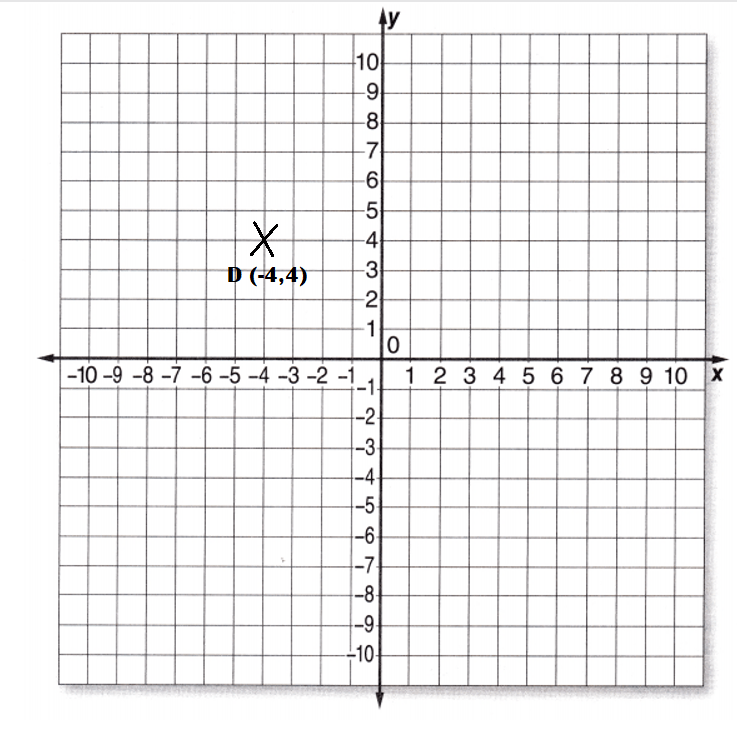Explanation:
D(-4, 4) represents D(x, y) axis points in the graph.

Question 5.
E(-4, 4)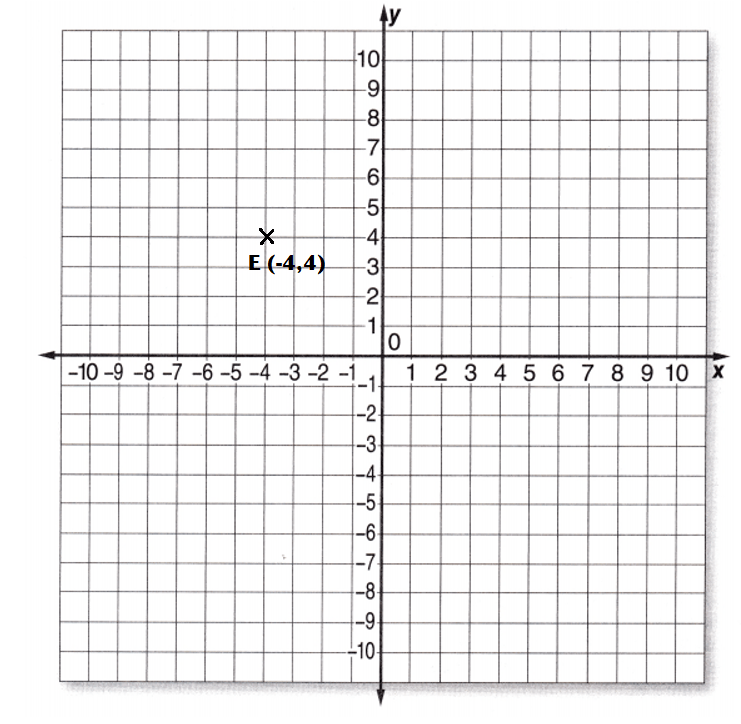Explanation:
E(-4, 4) represents E(x, y) axis points in the graph.

Question 6.
F(5, 2)Explanation:
F(5, 2) represents F(x, y) axis points in the graph.

Question 7.
G(8, 2)Explanation:
G(8, 2) represents G(x, y) axis points in the graph.

Question 8.
H(8, 6)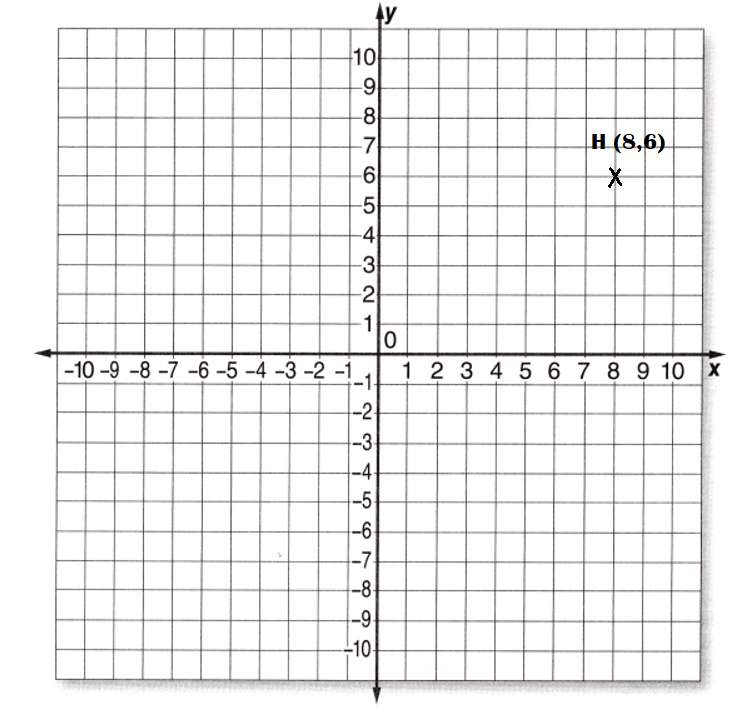Explanation:
H(8, 6) represents H(x, y) axis points in the graph.

Question 9.
J(-5, 6)Explanation:
J(-5, 6) represents J(x, y) axis points in the graph.

Question 10.
K(3, -4)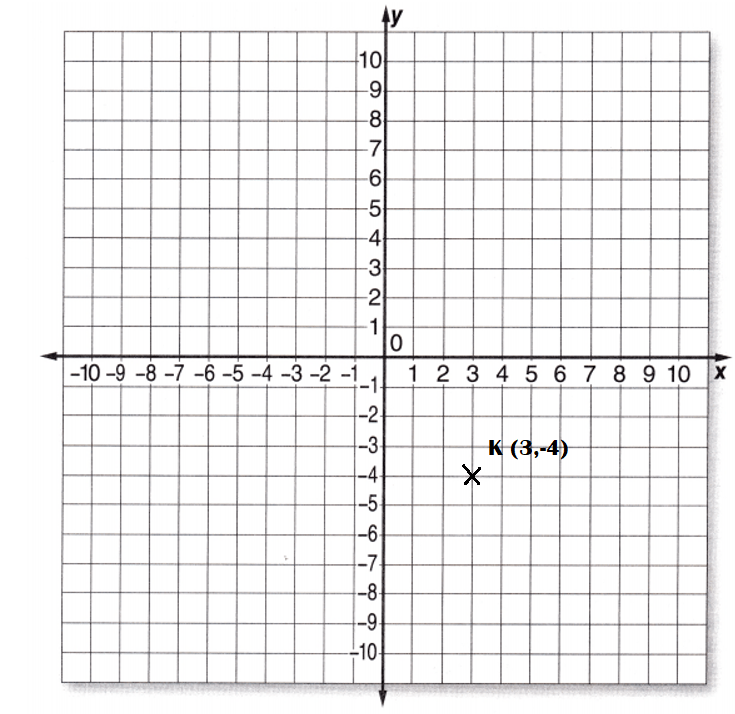Explanation:
K(3, -4)represents K(x, y) axis points in the graph.

Question 11.
L(-2, -2)